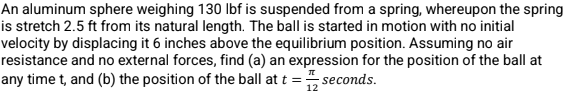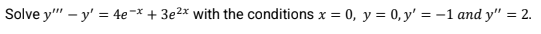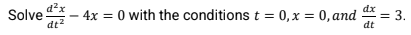# Second-Order Linear Equations Questions & Answers

Recent questions in Second order linear equationsAydin Welch 2022-12-20

### Find the general solution of the given differential equation:${y}^{″}-2{y}^{\prime }+y=0$blackdivcp 2022-10-23

### One solution of the different equation ${y}^{″}+{y}^{\prime }=0$ is $y={e}^{-x}$. Use Reduction of Order to find a second linearly independent solution.LEIDY KATERINE HERRERA AMORTEGUI 2022-08-11

### dx/dy=-(4y^2+6xy/3y^2+2xcapellitad9 2022-07-17

### If $y=x{e}^{7x}$ is a solution of a linear homogeneous 2nd order DE, then another solution might be?Sunita Jain 2022-06-12

### Determine the largest interval in which the initial value problem (t − 3)y ′′ − ty′ + 6y = sin t has unique solution. y(−3) = 2, y′ (−3) = 1Bacille John Purca 2022-05-18

###Bacille John Purca 2022-05-18

###Bacille John Purca 2022-05-18

###Amya Horn 2022-03-31

### Solve the differential equation by using method of variation of parametrs$y{}^{‴}+9{y}^{\prime }=\frac{1}{f\left(x\right)}$take $f\left(x\right)=\mathrm{cos}11x$wibawanyacl3q 2022-03-29

### Solve differential equation.$y{}^{″}+2{y}^{\prime }=3{x}^{2}+2x$Kendall Daniels 2022-03-28

### A nonhomogeneous second-order linear equation and a complementary function ${y}_{c}$ are given. Find a particular solution of the equation.Reuben Brennan 2022-03-25

### Find a second-order lincar equation for which $y\left(x\right)={c}_{1}{e}^{3x}+{c}_{2}{e}^{5x}+\mathrm{sin}\left(2x\right)$is the general solutionCoradossi7xod 2022-03-25

### Consider the following linear second-order homogeneous differential equation with constant coefficients and two initial conditionsBlackettyl2j 2022-03-25

### Solve the following linear second order homogeneous differential equationPizzadililehz 2022-03-25

### Find the general solution of the given differential equations$4y{}^{″}-4{y}^{\prime }-3=0$Destinee Hensley 2022-03-25

### Find the general solution of the given second-order differential equations$2y{}^{″}+2{y}^{\prime }+y=0$kembdumatxf 2022-03-25

### Find the general solution to the following second order differential equations.$y{}^{″}-{6}^{\prime }+25y=0$avalg10o 2022-03-25

### Find a particular solution to the differential equation.$y{}^{″}-{y}^{\prime }-6y=\mathrm{sin}t+3\mathrm{cos}t$Jackson Floyd 2022-03-25

### Find ODE having the given function as its general solution.$y={x}^{2}+{c}_{1}{e}^{2x}+{c}_{2}{e}^{3x}$ideklaraz7xz 2022-03-24

### Consider the following linear second order homogeneous differential equation wit constant coefficients and two initial conditionsSolve the differential equation.

Dealing with second-order linear equations is quite complex for post-secondary algebra students who have to provide such equations for statistical analysis where calculus is involved. It’s a reason why linear equation examples help with the system of linear equations, especially when it has to be implemented in Sociology, Engineering, or even Business Management. It is a reason why linear equations word problems have also been offered below as students cannot approach them without some linear equations solver that would contain an explanation with details. You can see answers to questions and learn how linear equations and inequalities can be solved.